# JP3873972B2 - Power supply device and method for creating switching signal for on / off control of switching element of converter section constituting power supply device - Google Patents

Power supply device and method for creating switching signal for on / off control of switching element of converter section constituting power supply device Download PDF

## Info

Publication number
JP3873972B2
JP3873972B2 JP2003542531A JP2003542531A JP3873972B2 JP 3873972 B2 JP3873972 B2 JP 3873972B2 JP 2003542531 A JP2003542531 A JP 2003542531A JP 2003542531 A JP2003542531 A JP 2003542531A JP 3873972 B2 JP3873972 B2 JP 3873972B2
Authority
JP
Japan
Prior art keywords
voltage
input
power supply
output
current
Prior art date
Legal status (The legal status is an assumption and is not a legal conclusion. Google has not performed a legal analysis and makes no representation as to the accuracy of the status listed.)
Active
Application number
JP2003542531A
Other languages
Japanese (ja)
Other versions
JPWO2003065560A1 (en
Inventor

Original Assignee

Priority date (The priority date is an assumption and is not a legal conclusion. Google has not performed a legal analysis and makes no representation as to the accuracy of the date listed.)
Filing date
Publication date
Application filed by 三菱電機株式会社 filed Critical 三菱電機株式会社
Priority to PCT/JP2002/000679 priority Critical patent/WO2003065560A1/en
Publication of JPWO2003065560A1 publication Critical patent/JPWO2003065560A1/en
Application granted granted Critical
Publication of JP3873972B2 publication Critical patent/JP3873972B2/en
Application status is Active legal-status Critical
Anticipated expiration legal-status Critical

## Images

•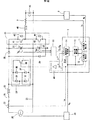••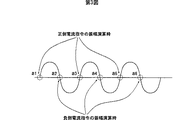•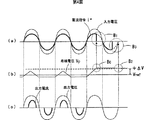•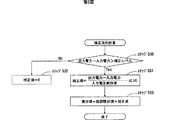•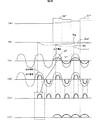•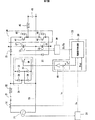•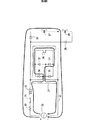•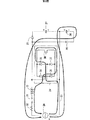•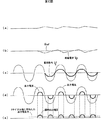•## Classifications

• HELECTRICITY
• H02GENERATION; CONVERSION OR DISTRIBUTION OF ELECTRIC POWER
• H02MAPPARATUS FOR CONVERSION BETWEEN AC AND AC, BETWEEN AC AND DC, OR BETWEEN DC AND DC, AND FOR USE WITH MAINS OR SIMILAR POWER SUPPLY SYSTEMS; CONVERSION OF DC OR AC INPUT POWER INTO SURGE OUTPUT POWER; CONTROL OR REGULATION THEREOF
• H02M7/00Conversion of ac power input into dc power output; Conversion of dc power input into ac power output
• H02M7/42Conversion of dc power input into ac power output without possibility of reversal
• H02M7/44Conversion of dc power input into ac power output without possibility of reversal by static converters
• H02M7/48Conversion of dc power input into ac power output without possibility of reversal by static converters using discharge tubes with control electrode or semiconductor devices with control electrode
• HELECTRICITY
• H02GENERATION; CONVERSION OR DISTRIBUTION OF ELECTRIC POWER
• H02MAPPARATUS FOR CONVERSION BETWEEN AC AND AC, BETWEEN AC AND DC, OR BETWEEN DC AND DC, AND FOR USE WITH MAINS OR SIMILAR POWER SUPPLY SYSTEMS; CONVERSION OF DC OR AC INPUT POWER INTO SURGE OUTPUT POWER; CONTROL OR REGULATION THEREOF
• H02M7/00Conversion of ac power input into dc power output; Conversion of dc power input into ac power output
• H02M7/42Conversion of dc power input into ac power output without possibility of reversal
• H02M7/44Conversion of dc power input into ac power output without possibility of reversal by static converters
• H02M7/48Conversion of dc power input into ac power output without possibility of reversal by static converters using discharge tubes with control electrode or semiconductor devices with control electrode
• H02M7/53Conversion of dc power input into ac power output without possibility of reversal by static converters using discharge tubes with control electrode or semiconductor devices with control electrode using devices of a triode or transistor type requiring continuous application of a control signal
• H02M7/537Conversion of dc power input into ac power output without possibility of reversal by static converters using discharge tubes with control electrode or semiconductor devices with control electrode using devices of a triode or transistor type requiring continuous application of a control signal using semiconductor devices only, e.g. single switched pulse inverters
• H02M7/538Conversion of dc power input into ac power output without possibility of reversal by static converters using discharge tubes with control electrode or semiconductor devices with control electrode using devices of a triode or transistor type requiring continuous application of a control signal using semiconductor devices only, e.g. single switched pulse inverters in a push-pull configuration
• H02M7/53803Conversion of dc power input into ac power output without possibility of reversal by static converters using discharge tubes with control electrode or semiconductor devices with control electrode using devices of a triode or transistor type requiring continuous application of a control signal using semiconductor devices only, e.g. single switched pulse inverters in a push-pull configuration with automatic control of output voltage or current
• HELECTRICITY
• H02GENERATION; CONVERSION OR DISTRIBUTION OF ELECTRIC POWER
• H02MAPPARATUS FOR CONVERSION BETWEEN AC AND AC, BETWEEN AC AND DC, OR BETWEEN DC AND DC, AND FOR USE WITH MAINS OR SIMILAR POWER SUPPLY SYSTEMS; CONVERSION OF DC OR AC INPUT POWER INTO SURGE OUTPUT POWER; CONTROL OR REGULATION THEREOF
• H02M1/00Details of apparatus for conversion
• H02M2001/0003Details of control, feedback and regulation circuits
• H02M2001/0016Control circuits providing compensation of output voltage deviations using feedforward of disturbance parameter
• H02M2001/0022Control circuits providing compensation of output voltage deviations using feedforward of disturbance parameter the disturbance parameter being input voltage fluctuations

## Description

The present invention relates to a power supply device and a method for creating a switching signal for controlling on / off of a switching element of a converter section constituting the power supply device.

FIG. 7 is a diagram showing the configuration of a conventional power supply apparatus.
In the figure, 20 is an AC power source, 21 is a switch for turning on and off the connection with the AC power source 20, 22 is a boosting reactor, and 23 is a common bus connecting one end of the AC power source 20 and one end of a load (not shown). is there. Also, 24 is an AC / DC conversion circuit, 25 and 26 are diodes, 27 is a capacitor connected between the positive electrode P and the common bus 23, 28 is a capacitor connected between the common bus 23 and the negative electrode N, and 29 is a DC / DC It is an inverter part as an AC conversion circuit.

Further, 30 is a bus voltage detection circuit for detecting the voltages of the capacitors 27 and 28, 31 is an input voltage detection circuit for detecting the voltage of the AC power supply 20, 32 is a current detection circuit for detecting the current of the AC power supply 20, and 33 is AC It is a control unit that controls the / DC conversion circuit 24.
Reference numerals 34, 35, 36, and 37 denote diodes, and 38 denotes a switching element. The diodes 34, 35, 36, 37 and the switching element 38 constitute an AC / DC conversion circuit 24. The AC / DC conversion circuit 24 and the reactor 22 constitute a boost chopper circuit.

Reference numeral 39 denotes a current command generator, and 40 denotes a comparator. A control unit 33 is configured by the current command generation unit 39 and the comparator 40.
Reference numerals 41 and 42 denote switching elements, and reference numerals 43 and 44 denote diodes connected to the switching elements 41 and 42 in antiparallel. The inverter elements 29 are configured by the switching elements 41 and 42 and the diodes 43 and 44.

Reference numeral 45 is a reactor, and 46 is a capacitor.
Va is an input voltage detection value detected by the input voltage detection circuit 31, Vref is a reference voltage command, VP and VN are bus voltage detection values output from the bus voltage detection circuit 30, and ia is a current detection circuit 32. The detected input current detection value, i 2 *, is a current command output from the current command creation unit 39.

8 and 9 are diagrams for explaining the operation of charging the capacitors 27 and 28 in the conventional power supply apparatus. In the figure, 20 to 28, 32, and 34 to 38 are the same as those in FIG.
The operation of the conventional power supply apparatus will be described with reference to FIGS.
In the conventional power supply device, the current command i 2 * is created from the reference voltage command Vref and the bus voltage detection value VP (or VN) output from the bus voltage detection circuit 30 by the current command creation means 39 of the controller 33. .

The comparator 40 of the control unit 33 compares the current command i2 * with the input current detection value ia detected by the current detection circuit 32. When the input current detection value ia exceeds the current command i2 *, switching is performed. When the switching signal for turning on / off the element 38 is turned off and the input current detection value ia falls below the current command i2 *, the switching signal for turning on / off the switching element 38 is turned on.

By switching on / off the switching element 38 of the AC / DC conversion circuit 24 with this switching signal, the AC power of the AC power source 20 is converted to DC by the path shown in FIG. 8 or 9 when the switching signal is turned on. While converting into electric power, it accumulates | stores energy in the reactor 22, and pressure | voltage-rises, and the capacitor | condensers 27 and 28 are charged with the sum of the energy accumulate | stored in the reactor 22 when the switching signal is OFF, and the alternating voltage of a power supply device.

By performing on / off control of the switching elements 41 and 42 of the inverter unit 29, the DC voltage charged in the capacitors 27 and 28 is converted into AC power of a predetermined voltage and output.
Next, the operation of charging the capacitor 27 when the AC power supply 20 is positive will be described with reference to FIG.

When the AC power supply 20 is positive, the control unit 33 turns on the switching element 38 of the AC / DC conversion circuit 24, whereby the AC power supply 20 → switch 21 → reactor 22 → diode 34 → switching element 38 → diode 37 → common. Energy is stored in the reactor 22 through a path of the bus 23 → the AC power supply 20. Subsequently, the control unit 33 turns off the switching element 38 and uses the energy accumulated in the reactor 22 through the path of the AC power supply 20 → the switch 21 → the reactor 22 → the diode 25 → the capacitor 27 → the common bus 23 → the AC power supply 20. The capacitor 27 is charged.

The operation of charging the capacitor 28 by the AC / DC conversion circuit 24 when the AC power supply 20 is negative will be described with reference to FIG.
When the AC power supply 20 is negative, the control unit 33 turns on the switching element 38 of the AC / DC conversion circuit 24, whereby the AC power supply 20 → the common bus 23 → the diode 35 → the switching element 38 → the diode 36 → the reactor 22 → Energy is stored in the reactor 22 through a path of the switch 21 → the AC power supply 20. Subsequently, the switching element 38 is turned off, and the capacitor 28 is charged by the energy accumulated in the reactor 22 through the path of the AC power supply 20 → the common bus 23 → the capacitor 28 → the diode 26 → the reactor 22 → the switch 21 → the AC power supply 20. To do.

FIGS. 10 and 11 are diagrams showing various waveforms in the conventional power supply apparatus. FIG. 10 shows a case where the load is light, and FIG. 11 shows a case where the load is increased. In the figure, (a) is a waveform of current command amplitude, (b) is a waveform of bus voltage, (c) is a waveform of input voltage and current command, (d) is a waveform of output current and output voltage, (e) Is the waveform of the instantaneous output power.
In the conventional power supply device, the voltage of the capacitors 27 and 28 is controlled to be held at a predetermined voltage by controlling the time for turning on / off the switching element 38 as described above.

Further, the switching signal to the switching element 38 is instantaneously controlled so that the bus voltage follows the reference voltage command. For this reason, even if the average load during a half cycle is constant, if the instantaneous load fluctuates greatly, the bus voltage will also fluctuate in synchronization with it, and if the response is made faster, the input current will also contain a small ripple. It will be. When the load is light, the fluctuation of the bus voltage is small (FIG. 10 (b)), but when the load is increased, a large ripple voltage is generated in the bus voltage (FIG. 11 (b)). .

The negative bus voltage VN is the same as the positive bus voltage VP, and the description thereof is omitted.
As described above, in the conventional power supply device, when the response is accelerated, when the load is increased, a large ripple voltage is generated in the bus voltage when the instantaneous load greatly fluctuates. There is a problem that it is necessary to use an element with a high breakdown voltage in consideration of the peak value of the ripple voltage of the bus voltage.

The present invention has been made to solve the above-described problems. A first object of the present invention is to control the bus voltage to the reference voltage while suppressing fluctuations in the bus voltage within a predetermined range regardless of the size of the load. A power supply device that can match the command is obtained.
The second object is to obtain a power supply device that can improve the load response and can stabilize the bus voltage even in an impact load.

A power supply device according to the present invention includes a diode bridge and a switching element, and converts a AC power into a DC power, a boosting reactor constituting the converter section and a boosting chopper circuit, and the boosting chopper circuit boosts the power. An input current for detecting an input current of an AC power supply in a power supply device having a capacitor for charging a DC voltage, a control circuit for controlling on / off of the switching element, and an inverter unit for converting DC power to AC power A detection circuit; an input voltage detection unit that detects an input voltage of an AC power supply; and a DC voltage detection circuit that detects a voltage of the capacitor. The control circuit changes a sign of an input voltage from the AC power supply. At the time when the AC power supply is half-powered by PI control based on the difference between the reference voltage command and the voltage of the capacitor. While calculating the amplitude of the current command for each vehicle, calculate the normalized current command by dividing the amplitude of this current command by the peak value of the previous input voltage and multiplying by the input voltage from the AC power supply, By comparing this current command with the input current detected by the input current detection circuit, a switching signal for on / off control of the switching element is created. Therefore, every half cycle of the input voltage from the AC power supply Based on the amplitude of the current command obtained by PI control, a current command similar to the input voltage can be created, and the bus voltage can be controlled while keeping the fluctuation of the bus voltage within a specified range regardless of the load size. It is possible to obtain a power supply device that can match the voltage command.

In addition, when the difference between the reference voltage command and the voltage of the capacitor detected by the DC voltage detection circuit exceeds a predetermined allowable value, the control circuit determines the amplitude of the current command calculated by the PI control. Since the integral term is cleared to 0, it is possible to obtain a power supply device that can suppress an increase in bus voltage even when the impact load is lost or when the magnitude of the input voltage changes suddenly.

Further, an output current detection circuit that detects an output current of the inverter unit, an output voltage detection unit that detects an output voltage of the inverter unit, an output current from the current detection circuit, and an output voltage from the output voltage detection unit An output power detection unit that calculates output power, and an input power detection unit that inputs input current from the input current detection circuit and input voltage from the input voltage detection circuit and calculates input power, and the control The circuit calculates a correction term by dividing the difference between the output power and the input power by the effective value of the input voltage when the difference between the output power and the input power exceeds a predetermined value, and an integral term of the PI control. Therefore, it is possible to obtain a power supply apparatus capable of instantaneously improving the response of the current command and stabilizing the bus voltage even in an impact load.

The switching signal generation method in the power supply device of the present invention includes a diode bridge and a switching element, and a switching signal for on / off control of the switching element of the converter unit that converts AC power from the AC power source into DC power. In the production method, at the time when the sign of the input voltage from the AC power supply changes, based on the difference between the reference voltage command and the voltage of the capacitor, the amplitude of the current command is set for each half cycle of the input voltage by PI control. And calculating the difference between the output power and the input power when the difference between the output power from the inverter unit that converts DC power into AC power and the input power from the AC power source exceeds a predetermined value. Calculate the correction term by dividing by the effective value of the input voltage, add it to the integral term of the PI control, and input the amplitude of this current command last time A step of calculating a normalized current command by dividing by the peak value of the voltage and further multiplying by the input voltage from the AC power supply, and comparing the current command with the input current detected by the input current detection circuit Thus, the step of creating a switching signal for controlling on / off of the switching element is provided, so that the bus voltage can be controlled stably.

Embodiment 1 FIG.
FIG. 1 is a diagram showing a configuration of a power supply apparatus according to Embodiment 1 of the present invention. In the figure, 20 to 32, 34 to 37, 40 and 41 to 46 are the same as those in FIG.
Reference numeral 1 denotes a control unit, 2 denotes an output current detection circuit that detects an output current of the inverter unit 29, and 3 denotes an output voltage detection unit that detects an output voltage of the inverter unit 29. Reference numeral 11 denotes an output power detection unit that inputs output current from the output current detection circuit 2 and output voltage from the output voltage detection unit 3 to calculate output power. Reference numeral 12 denotes an input voltage and current detection circuit 32 from the input voltage detection circuit 31. Are an input power detection unit that calculates input power, 13 is a correction term calculation unit, and 14 is a current command generation unit.

Further, Va is an input voltage detection value detected by the input voltage detection circuit 31, Vref is a reference voltage command, VP and VN are bus voltage detection values output from the bus voltage detection circuit 30, and ia is detected by the current detection circuit 32. The input current detection value, Vb is the output voltage detection value output from the output voltage detection unit 3, ib is the output current detection value output from the current detection circuit 2, and i1 * is output from the current command generation unit 14. Current command.

The current command generation unit 14 generates a current command based on the reference voltage command Vref, the input voltage detection value Va detected by the input voltage detection circuit 31, and the bus voltage detection value VP (or VN) detected by the bus voltage detection circuit 30. i1 * is created and amplified to the same range as the input current detection value ia by an amplifier (not shown). The comparator 40 compares a difference between the amplified current command i1 * and the input current detection value ia detected by the current detection circuit 32, and determines a switching signal for turning on / off the gate of the switching element 38.

FIG. 2 is a flowchart of a current command generation process in the power supply apparatus according to Embodiment 1 of the present invention. In the figure, steps S1 to S15 are current command amplitude calculation processes, and steps S20 to S27 are current command calculation processes.
FIG. 3 is a diagram for explaining the relationship between the waveform of the input voltage and the amplitude calculation of the current command in the power supply device according to Embodiment 1 of the present invention. In the figure, a1, a3, and a5 are times when the amplitude of the positive current command is calculated, and a2, a4, and a6 are times when the amplitude of the negative current command is calculated.

FIG. 4 is a diagram showing the relationship between the input voltage and the current command in the power supply apparatus according to Embodiment 1 of the present invention, where (a) is the relationship between the input voltage and the current command, and (b) is the diagram. The relationship between the reference voltage command and the positive bus voltage VP, (c) is the relationship between the output current and the output voltage. In the figure, when the instantaneous value VP of the bus voltage exceeds the allowable range (Vref + ΔV) of the reference voltage command (point B0 in FIG. 4 (b)), the amplitude I * of the current command is set to 0 in the half cycle. This is an example in which (B1 point in FIG. 4 (a)) is made. The negative bus voltage VN is the same as the positive bus voltage VP, and the description thereof is omitted.

A current command generation process in the power supply apparatus according to Embodiment 1 of the present invention will be described with reference to FIGS.
In step S <b> 1, the current command generator 14 determines the input voltage detection value Va input from the input voltage detection circuit 31. The previously read input voltage detection value (hereinafter referred to as the previous input voltage) is compared with the current input voltage detection value (hereinafter referred to as the current input voltage), and the previous input voltage <0 and the current input voltage ≧ 0. If not, the process jumps to step S11.

If it is determined in step S1 that the previous input voltage <0 and the current input voltage ≧ 0 (in FIG. 3, the input voltage detection value Va is a1, a3, and a5 when the negative side becomes the positive side), the step S2 After calculation processing of the correction term shown in FIG. 5 to be described later, in step S3, based on the reference voltage command Vref and the values V to P obtained by removing the noise after the first-order lag filter of the bus voltage detection value, an expression is obtained by PI control. The amplitude I * of the current command is created in (1).

I * = Kp (Vref−V˜P) + ΣKi (Vref−V˜P) (1)
However, Kp is a proportional gain and Ki is an integral gain. In step S4, the instantaneous value VP of the bus voltage is compared with the reference voltage command Vref, and if VP ≦ Vref + ΔV, the process jumps to step S11. Here, ΔV is an allowable level.
If it is determined in step S4 that VP> Vref + ΔV (B2 in FIG. 4 (b)), the amplitude I * of the current command created in equation (1) in step S3 is cleared to 0 in step S5 (FIG. 4). Along with B3) in (a), the integral term of the second term on the right side of Equation (1) is cleared to 0, and the process proceeds to step S11.

In step S11, the previous input voltage is compared with the current input voltage. If the previous input voltage ≧ 0 and the current input voltage <0 is not satisfied, the process jumps to step S20.
If it is determined in step S11 that the previous input voltage ≧ 0 and the current input voltage <0 (in FIG. 3, the input voltage changes from the positive side to the negative side a2, a4, and a6), step S12 will be described later. After calculation processing of the correction term shown in FIG. 5 , in step S13, the reference voltage command Vref and the values V to N after the first-order lag filter of the bus voltage detection value are used to perform PI control according to the equation (2). Create the amplitude I * of the current command.

I * = Kp (Vref−V˜N) + ΣKi (Vref−V˜N) (2)
However, Kp is a proportional gain and Ki is an integral gain. In step S14, the instantaneous value VN of the bus voltage is compared with the reference voltage command Vref. If VN ≦ Vref + ΔV, the process jumps to step S20. Here, ΔV is an allowable level.

If it is determined in step S14 that VN> Vref + ΔV, the amplitude I * of the current command created in equation (2) in step S13 is cleared to 0 in step S15, and the integral term of the second term on the right side of equation (2) is set. Is cleared to 0, and the process proceeds to step S20.
The current command generation unit 14 in the power supply device according to Embodiment 1 of the present invention is configured such that the current on the positive side at the time when the input voltage is switched from the negative side to the positive side (a1, a3, a5 in FIG. 3 (a)). The amplitude of the command is calculated (step S3), and the amplitude of the negative current command is calculated when the input voltage is switched from the positive side to the negative side (a2, a4, a6 in FIG. 3A) ( As shown in step S13), a current command is created when the sign of the input voltage changes twice in one cycle of the input voltage (FIG. 3 (a)).

In steady state, the average power of each positive and negative load is constant. By calculating the amplitude I * of the current command every half cycle, the stable current that eliminates the effects of load fluctuations that change during the half cycle is removed. The command amplitude I * can be created, and the bus voltage can be matched with the reference voltage command while suppressing the variation of the bus voltage within a predetermined range regardless of the load.

Steps S4 and S14 check whether the instantaneous values VP and VN of the bus voltage are within the allowable range (Vref + ΔV) of the reference voltage command. The instantaneous values VP and VN of the bus voltage are the allowable range of the reference voltage command. Is exceeded (VP> Vref + .DELTA.V, VN> Vref + .DELTA.V), the amplitude I * and integral term of the current command obtained by the equation (1) or (2) are cleared to 0 in steps S5 and S15.

When the bus voltage rises significantly, the rise of the bus voltage can be suppressed by setting the current command amplitude I * to 0 and turning off the switching element 38.
In step S20, whether the sign of the input voltage is positive or negative is determined. If the input voltage is negative, the process jumps to step S24.
If it is determined in step S20 that the input voltage is on the positive side, the positive peak value of the input voltage is calculated in step S21.

In step S22, the instantaneous value VP of the bus voltage is compared with the reference voltage command Vref, and when the allowable level is ΔV, if VP ≦ Vref + ΔV, the process jumps to step S27.
If VP> Vref + ΔV in step S22, the amplitude I * of the current command created in equation (1) is set to 0 in step S23, the process proceeds to step S27, and a current command i * is created.
If it is determined in step S20 that the input voltage is negative, a negative peak value of the input voltage is calculated in step S24.

In step S25, the instantaneous value VN of the bus voltage is compared with the reference voltage command Vref, and when the allowable level is ΔV, if VN ≦ Vref + ΔV, the process jumps to step S27.
If VN> Vref + ΔV in step S25, the amplitude I * of the current command created in equation (2) is set to 0 in step S26, and the process proceeds to step S27 to create a current command i *.
In step S27, the current command i * is created by normalizing the input voltage instantaneous value / previous input voltage peak value Vpeak according to the equation (3).

i * = (Instantaneous input voltage / Peak value of previous input voltage) × I * (3)
In equation (3), since I * and Vpeak are fixed values during a half cycle, a current command i * proportional to the input voltage can be created, and a current command i * with an input power factor of 1 and can do.

In step S22, step S23, step S25, and step S26, when the instantaneous values VP and VN of the bus voltage exceed the allowable range (Vref + ΔV) of the reference voltage command, the half cycle sets the amplitude I * of the current command to 0. Thus, even when the impact load is lost or when the magnitude of the input voltage changes suddenly, an increase in the bus voltage can be suppressed.

Here, when the integral term is cleared when the instantaneous value VP, VN of the bus voltage exceeds the allowable range (Vref + ΔV) of the reference voltage command due to the fluctuation of the input voltage or the load, the amplitude of the current command increases again. In order to prevent an increase in bus voltage fluctuation, it is assumed that the integral term is not cleared in steps S23 and S26. Accordingly, the timing for clearing the integral term is only the processing (steps S5 and S15 in FIG. 2) when the input voltage indicated by a1 to a6 in FIG.

FIG. 5 is a flowchart of correction term calculation processing in the power supply apparatus according to Embodiment 1 of the present invention, and is a detailed flowchart of correction term calculation in steps S2 and S12 of FIG.

FIG. 6 is a diagram for explaining the relationship between the waveform of the input voltage and the amplitude calculation of the current command in the power supply apparatus according to Embodiment 1 of the present invention, where (a) is the waveform of the amplitude of the current command, and (b). (C) is the waveform of the input voltage and current command, (d) is the waveform of the output voltage and output current, (e) is the waveform of output power, and (f) is the waveform of input power.
In the figure, I0 * is the amplitude of the current command with correction processing, I1 * is the amplitude of the current command without correction processing, i0 * is the current command with correction processing, i1 * is the current command without correction processing, and Vref is the reference. The voltage command, VP0 is a bus voltage with correction processing, and VP1 is a bus voltage without correction processing.

The correction term processing at the time of creating the current command in the power supply device according to Embodiment 1 of the present invention will be described with reference to FIGS. 2, 5, and 6. FIG.
The correction term calculation unit 13 detects when the input voltage is switched from the negative side to the positive side (a1, a3, a5 in FIG. 3A) or when the input voltage is switched from the positive side to the negative side (FIG. 3). In (a) a2, a4, a6), the correction term shown in FIG. 5 is calculated.

In FIG. 5, in step S30, the input power Win output from the input power detection unit 12 is compared with the output power Wout output from the output power detection unit 11, and the difference between the output power and the input power is set to a correction level. If exceeded, in step S31, the drop in the bus voltage due to the impact load is calculated as a correction term using equation (4), and the process proceeds to step S33.
Correction term = √2 × (Wout−Win) / Vrms Expression (4)
Here, Vrms is an input voltage effective value.

If it is determined in step S30 that the difference between the output power and the input power is not more than the correction level, the correction term is cleared to 0 in step S32, and the process proceeds to step S33.
In step S33, the correction term calculated in step S31 or step S32 described above is added to the previous integral term in equation (1) or equation (2) for calculating the amplitude of the current command.
When the proportional gain Kp and integral gain Ki in the formula (1) or formula (2) for calculating the amplitude I * of the current command by the PI control are set to gains that are stable at a constant load, the gain is increased when an impact load is applied. Therefore, after the bus voltage is greatly reduced as indicated by VP1 in FIG. 6 (b), the integral term increases, and the amplitude I * of the current command gradually increases as indicated by I1 * in FIG. 6 (a). Since it becomes larger, the bus voltage recovery operation is delayed.

Also, by lowering the proportional gain Kp and integral gain Ki above the gain that stabilizes at a constant load, and improving the response of the amplitude I * of the current command when an impact load is applied, the reduction of the bus voltage when the impact load is applied is suppressed. However, if the responsiveness is increased too much, the bus voltage tends to vibrate, making it difficult to adjust the gain optimally for the system in consideration of the impact load.

As shown in FIG. 5 above, when the difference between the output power and the input power exceeds the correction level, the decrease in the bus voltage due to the impact load is calculated as a correction term, and the equation (1) or ( Since the integral term in 2) is added, the amplitude of the current command can be increased instantaneously, and the proportional gain Kp in the PI control shown in the equation (1) or (2) can be increased even at the time of impact load. With the integral gain Ki set to a gain that is stable at a constant load, the load response can be improved, and the bus voltage drop can be suppressed.

Switching between load fluctuations, power fluctuations, and control (back-up operation in which the bus voltage is boosted from a battery (not shown) and bypass operation in which the input power is output as it is via a relay is switched to the above-mentioned operation in which the voltage is boosted by AC power input. In order to improve the response until the integral term of the PI control for obtaining the amplitude of the current command increases, the shortage of the current command is calculated from the difference between the input power and the output power, and the integral term of the PI control Since correction is performed as a correction term, it is possible to minimize a decrease in bus voltage.

By the way, although the example in which the output voltage is synchronized with the input voltage has been described in the above description, the processing for performing the calculation every 0 crosses of the input voltage has the same effect even when the output voltage is not synchronized with the input voltage.

As described above, the switching signal generation method for on / off control of the switching element of the converter unit constituting the power supply device or the power supply device according to the present invention includes load fluctuation, power supply fluctuation, control switching (battery Even when switching from backup operation for boosting the bus voltage (not shown) or bypass operation in which the input power is directly output by the relay to operation for boosting by the AC power input described above, the fluctuation of the bus voltage is within a predetermined range. It is suitable for an uninterruptible power supply that supplies AC power to a load during a momentary power failure.

FIG. 1 is a diagram showing a configuration of a power supply apparatus according to Embodiment 1 of the present invention.
FIG. 2 is a flowchart of a current command generation process in the power supply apparatus according to Embodiment 1 of the present invention.
FIG. 3 is a diagram for explaining the relationship between the waveform of the input voltage and the amplitude of the current command in the power supply device according to Embodiment 1 of the present invention.
FIG. 4 is a diagram showing the relationship between the input voltage and the current command in the power supply device according to Embodiment 1 of the present invention.
FIG. 5 is a flowchart of correction term calculation processing in the power supply apparatus according to Embodiment 1 of the present invention.
FIG. 6 is a diagram for explaining the relationship between the waveform of the input voltage and the amplitude calculation of the current command in the power supply device according to Embodiment 1 of the present invention.
FIG. 7 is a diagram showing the configuration of a conventional power supply apparatus.
FIG. 8 is a diagram for explaining the operation of charging the capacitor 27 in the conventional power supply apparatus (when the AC power supply 20 is positive).
FIG. 9 is a diagram for explaining the operation of charging the capacitor 27 in the conventional power supply device (when the AC power supply 20 is negative).
FIG. 10 is a diagram showing various waveforms in a conventional power supply device (when the load is light).
FIG. 11 is a diagram showing various waveforms in the conventional power supply device (when the load is increased).

## Claims (4)

1. A converter unit having a diode bridge and a switching element, and converting AC power into DC power;
A boosting reactor constituting the converter unit and the boosting chopper circuit;
A capacitor for charging a DC voltage boosted by the boost chopper circuit;
A control circuit for controlling on / off of the switching element;
An inverter unit for converting DC power into AC power;
In a power supply device having
An input current detection circuit for detecting the input current of the AC power supply;
An input voltage detector for detecting the input voltage of the AC power supply;
A DC voltage detection circuit for detecting the voltage of the capacitor,
The control circuit includes:
At the time when the sign of the input voltage from the AC power source changes, based on the difference between the reference voltage command and the voltage of the capacitor, the PI control calculates the amplitude of the current command every half cycle of the AC power source,
Divide the amplitude of this current command by the peak value of the previous input voltage, and further calculate the normalized current command by multiplying by the input voltage from the AC power source,
A power supply device characterized in that a switching signal for on / off control of the switching element is created by comparing the current command with an input current detected by the input current detection circuit.
2. The control circuit clears the amplitude and integral term of the current command calculated by the PI control to zero when the difference between the reference voltage command and the capacitor voltage detected by the DC voltage detection circuit exceeds a predetermined allowable value. The power supply apparatus according to claim 1, wherein the power supply apparatus is configured as described above .
3. An output current detection circuit for detecting an output current of the inverter unit;
An output voltage detection unit for detecting an output voltage of the inverter unit;
An output power detection unit that calculates an output power by inputting an output current from the output current detection circuit and an output voltage from the output voltage detection unit;
An input power detection unit that inputs an input current from the input current detection circuit and an input voltage from the input voltage detection circuit, and calculates an input power;
With
The control circuit includes:
When the difference between the output power and the input power exceeds a predetermined value, a correction term is calculated by dividing the difference between the output power and the input power by the effective value of the input voltage and added to the integral term of the PI control. The power supply device according to claim 1, which is configured as described above .
4. In a method of creating a switching signal that has a diode bridge and a switching element, and that controls on / off of the switching element of the converter unit that converts AC power from an AC power source into DC power.
Calculating the amplitude of the current command for each half cycle of the input voltage by PI control based on the difference between the reference voltage command and the voltage of the capacitor at the time when the sign of the input voltage from the AC power supply changes;
When the difference between the output power from the inverter unit that converts DC power into AC power and the input power from the AC power source exceeds a predetermined value, the difference between the output power and the input power is expressed as an effective value of the input voltage. Dividing to calculate a correction term and adding it to the integral term of the PI control;
Dividing the amplitude of this current command by the peak value of the previous input voltage, and further calculating the normalized current command by multiplying by the input voltage from the AC power source;
Creating a switching signal for controlling on / off of the switching element by comparing the current command with the input current detected by the input current detection circuit;
A method for creating a switching signal , comprising :
JP2003542531A 2002-01-30 2002-01-30 Power supply device and method for creating switching signal for on / off control of switching element of converter section constituting power supply device Active JP3873972B2 (en)

## Priority Applications (1)

Application Number Priority Date Filing Date Title
PCT/JP2002/000679 WO2003065560A1 (en) 2002-01-30 2002-01-30 Power supply and method for generating switching signal for turning on/off switching element of converter unit constituting the power supply

## Publications (2)

Publication Number Publication Date
JPWO2003065560A1 JPWO2003065560A1 (en) 2005-05-26
JP3873972B2 true JP3873972B2 (en) 2007-01-31

# Family

## Family Applications (1)

Application Number Title Priority Date Filing Date
JP2003542531A Active JP3873972B2 (en) 2002-01-30 2002-01-30 Power supply device and method for creating switching signal for on / off control of switching element of converter section constituting power supply device

## Country Status (3)

US (1) US6937488B2 (en)
JP (1) JP3873972B2 (en)
WO (1) WO2003065560A1 (en)

## Families Citing this family (14)

* Cited by examiner, † Cited by third party
Publication number Priority date Publication date Assignee Title
JP3980005B2 (en) * 2003-03-28 2007-09-19 松下電器産業株式会社 Inverter control device for motor drive and air conditioner
JP3874291B2 (en) * 2004-02-12 2007-01-31 Ｔｄｋ株式会社 Power supply
JP4461877B2 (en) * 2004-03-31 2010-05-12 株式会社安川電機 Inverter device
US7184282B2 (en) * 2005-03-11 2007-02-27 Origin Electric Company, Limited Single-phase power conversion device and three-phase power conversion device
JP4365376B2 (en) * 2006-02-14 2009-11-18 三菱電機株式会社 power converter
US7453241B2 (en) * 2006-11-29 2008-11-18 Sunpower, Inc. Electronic controller matching engine power to alternator power and maintaining engine frequency for a free-piston stirling engine driving a linear alternator
GB0710057D0 (en) * 2007-05-25 2007-07-04 Splashpower Power system
JP4637148B2 (en) * 2007-08-27 2011-02-23 日立アプライアンス株式会社 Power converter
US8054599B2 (en) * 2008-07-14 2011-11-08 International Business Machines Corporation Apparatus, system, and method for detecting a power system component failure
PL2284855T3 (en) * 2009-08-10 2017-09-29 Siemens Aktiengesellschaft Method for function testing a vacuum switch of a traction inverter
JP5580147B2 (en) * 2010-08-31 2014-08-27 オリジン電気株式会社 Stabilization control method
US8558522B2 (en) * 2010-12-18 2013-10-15 Semiconductor Components Industries, Llc Method for scaling a drive signal and circuit therefor
TWI513171B (en) * 2011-10-13 2015-12-11 Delta Electronics Inc Integrated inverter apparatus based on operating at optimal efficiency and method of operating the same
CN103218007B (en) * 2013-04-12 2015-04-08 刘昇澔 Circuit system and control method for electronic converter

## Family Cites Families (7)

* Cited by examiner, † Cited by third party
Publication number Priority date Publication date Assignee Title
EP0358191B1 (en) * 1988-09-06 1995-04-05 Kabushiki Kaisha Toshiba PWM-controlled power supply capable of eliminating modulation-frequency signal components from ground potentials
FR2713030B1 (en) * 1993-11-24 1996-01-12 Merlin Gerin The uninterruptible power supply through neutral, having a double step-up chopper.
JP3589086B2 (en) * 1999-05-17 2004-11-17 松下電器産業株式会社 Power supply
JP3324645B2 (en) * 1999-06-23 2002-09-17 サンケン電気株式会社 AC - DC converter
JP2001086737A (en) * 1999-09-10 2001-03-30 Yuasa Corp Power supply
JP2001095262A (en) 1999-09-24 2001-04-06 Mitsubishi Heavy Ind Ltd Dc-voltage controller
JP2002028853A (en) * 2000-07-14 2002-01-29 Ebara Corp Polishing device

## Also Published As

Publication number Publication date
JPWO2003065560A1 (en) 2005-05-26
WO2003065560A1 (en) 2003-08-07
US6937488B2 (en) 2005-08-30
US20040252533A1 (en) 2004-12-16

## Similar Documents

Publication Publication Date Title
US6215287B1 (en) Power supply apparatus
US20050269997A1 (en) Switching power source apparatus and power factor corrector
EP2299577B1 (en) Digital slope compensation for current mode control
JP3988724B2 (en) Power factor improving converter and control method thereof
US7615891B2 (en) Systems for and methods of controlling operation of a UPS
JP5104947B2 (en) Switching power supply
TWI472124B (en) Systems and methods for uninterruptible power supply control and system for distributing power to a load and computer readable medium thereof
JP4457692B2 (en) Maximum power tracking control method and power conversion device
JP2010114993A (en) Control system of power factor correction circuit
JP5163283B2 (en) Power factor correction circuit
WO2011030640A1 (en) Pfc converter
JP2006288156A (en) Dc-dc converter
US8184456B1 (en) Adaptive power converter and related circuitry
US20100109626A1 (en) Power factor correction power supply unit, and control circuit and control method used in the same
JP5267589B2 (en) Power converter
WO2008013353A1 (en) An controlling apparatus of a power converter of single-phase current for photovoltaic generation system
EP0780750A2 (en) Inventer control method and inventer apparatus using the method
KR100763135B1 (en) Photovoltaic power generation system and control method thereof
JP2006074965A (en) Power supply device
JP4706361B2 (en) System stabilization device
CN1521930A (en) Switch electrical source device
JP4512145B2 (en) Motor control device
DE112006002495T5 (en) Power factor correction digital controller and AC-to-DC power supply having the same
US7532491B2 (en) Apparatus and method for supplying DC power source
US6977830B2 (en) Power supply apparatus

## Legal Events

Date Code Title Description
A131 Notification of reasons for refusal

Free format text: JAPANESE INTERMEDIATE CODE: A131

Effective date: 20060718

A521 Written amendment

Free format text: JAPANESE INTERMEDIATE CODE: A523

Effective date: 20060809

A521 Written amendment

Free format text: JAPANESE INTERMEDIATE CODE: A523

Effective date: 20060821

TRDD Decision of grant or rejection written
A01 Written decision to grant a patent or to grant a registration (utility model)

Free format text: JAPANESE INTERMEDIATE CODE: A01

Effective date: 20061003

R151 Written notification of patent or utility model registration

Ref document number: 3873972

Country of ref document: JP

Free format text: JAPANESE INTERMEDIATE CODE: R151

A61 First payment of annual fees (during grant procedure)

Free format text: JAPANESE INTERMEDIATE CODE: A61

Effective date: 20061016

FPAY Renewal fee payment (event date is renewal date of database)

Free format text: PAYMENT UNTIL: 20091102

Year of fee payment: 3

FPAY Renewal fee payment (event date is renewal date of database)

Free format text: PAYMENT UNTIL: 20101102

Year of fee payment: 4

FPAY Renewal fee payment (event date is renewal date of database)

Free format text: PAYMENT UNTIL: 20111102

Year of fee payment: 5

FPAY Renewal fee payment (event date is renewal date of database)

Free format text: PAYMENT UNTIL: 20121102

Year of fee payment: 6

FPAY Renewal fee payment (event date is renewal date of database)

Free format text: PAYMENT UNTIL: 20121102

Year of fee payment: 6

FPAY Renewal fee payment (event date is renewal date of database)

Free format text: PAYMENT UNTIL: 20131102

Year of fee payment: 7

R250 Receipt of annual fees

Free format text: JAPANESE INTERMEDIATE CODE: R250

R250 Receipt of annual fees

Free format text: JAPANESE INTERMEDIATE CODE: R250

R250 Receipt of annual fees

Free format text: JAPANESE INTERMEDIATE CODE: R250

R250 Receipt of annual fees

Free format text: JAPANESE INTERMEDIATE CODE: R250

R250 Receipt of annual fees

Free format text: JAPANESE INTERMEDIATE CODE: R250